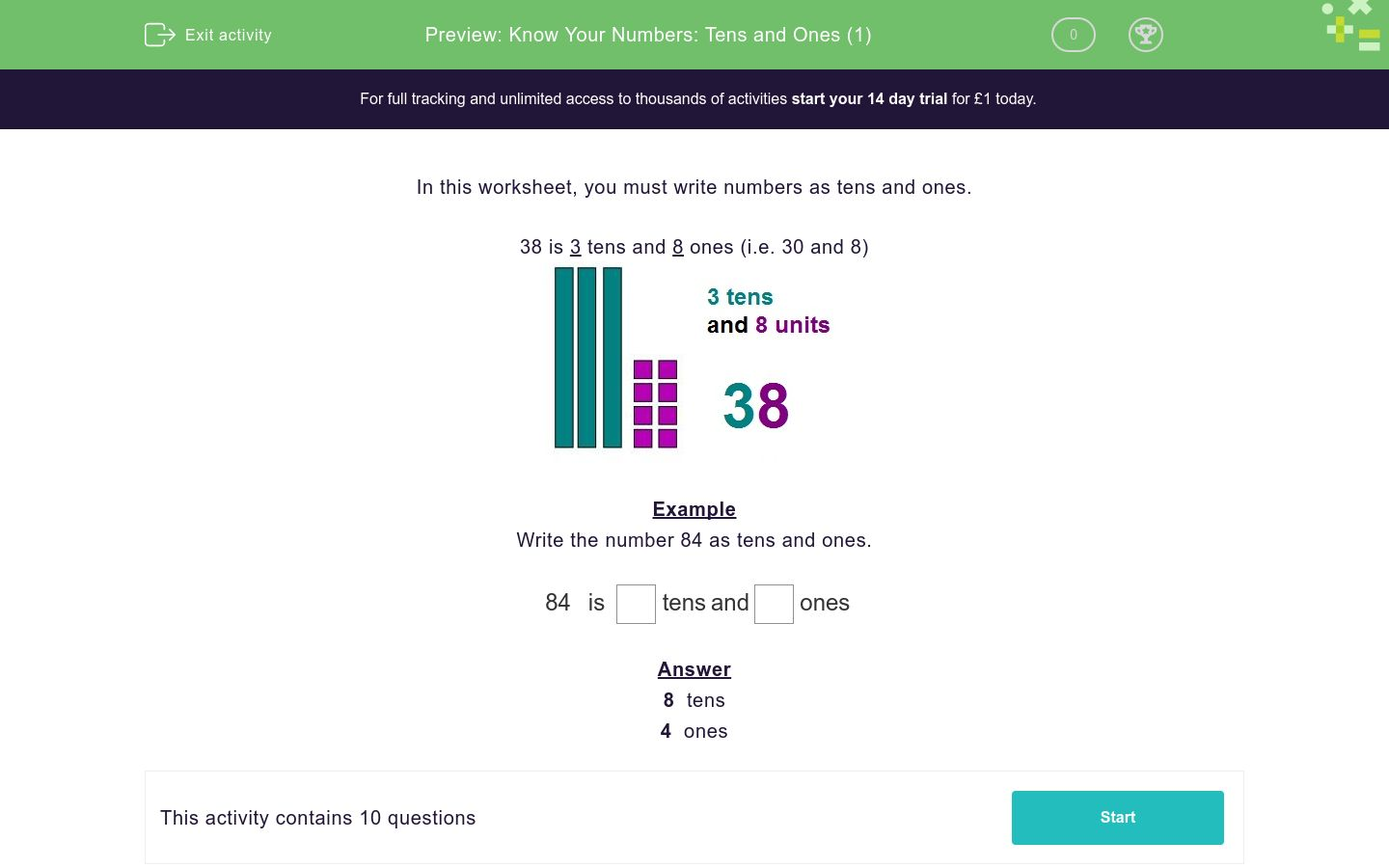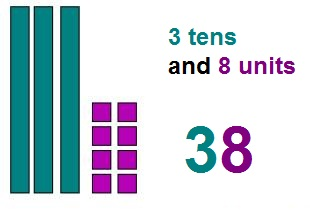# Know Your Numbers: Tens and Ones (1)

In this worksheet, students correctly select which part of the number is 'tens' and which part is 'ones'.Key stage:  KS 1

Curriculum topic:   Number: Number and Place Value

Curriculum subtopic:   Recognise Tens and Ones

Difficulty level:#### Worksheet Overview

In this worksheet, you must write numbers as tens and ones.

38 is 3 tens and 8 ones (i.e. 30 and 8)Example

Write the number 84 as tens and ones.

 84 is tens and ones